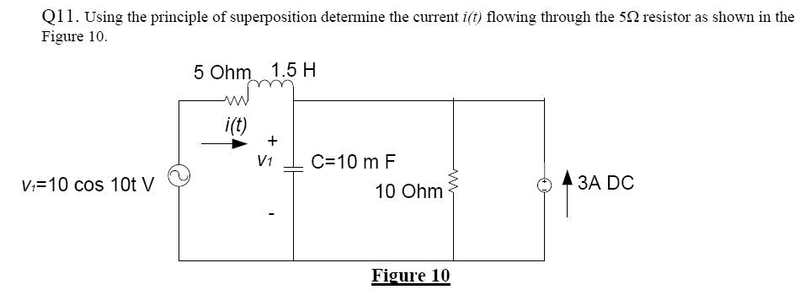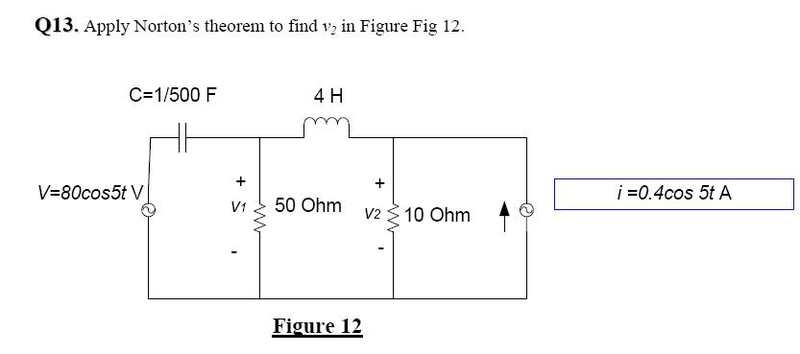# Quick phasor current question

## Homework StatementThe frequency is 50Hz (we've been told to always assume in our class that the frequency is 50Hz) and thus omega is 100pi.

## The Attempt at a Solution

I don't have time to scan an image of my attempt (complete attempt) however what my query is: the way i went about to answer this question, i firstly zeroed the current source, then i used the voltage divider to find the voltage across the 5ohm resistor, after this i used ohm's law (I=V/R) to find the current, which is (according to my calcuations): 0.021e^(-j1.56) Amps.
I then zeroed the voltage source, and used the current divider to find the current across the 5ohm resister, which was found to be: 0.0318e^(-j1.53) Amps. Adding these two (well i added their rectangular forms then converted back to exponential form), since that's what the superposition rule requires (right?), i get: 0.05e^(-j1.54) Amps.

I'm not too bothered whether anyone checks if this is right, but does the procedure seem right? As in, is it ok that i used the voltage divider, I=V/R then current divider? It seems rather simple to just use those three concepts/rules..it was my first attempt and that's what stood out. However someone i know in the same class went about it some other way (i don't know how, we exchanged text messages), and they ended up getting some 97Amps, which is kinda high i thought for such a small resistor value and even voltage value..thanks.

edit: i think i used the current divider incorrectly, the way i did it was: I = (Z(R 5ohm) / Z(R) + Z(L) + Z(C) + Z(R 10ohm)) * Isource. With Z being the impedances of the respective components..? I think i should have summed the inductor, capacitor and resistor(10ohm) and put that sum on the numerator? Then divide by the 5ohm resistor and summed impedances..

Last edited:

vela
Staff Emeritus
Homework Helper
Your basic strategy is correct, but I'm left wondering if you executed it correctly. You definitely made a mistake with the current divider, and your proposed alternative is wrong as well. What calculation did you do for the voltage divider?

^^I thought that's how you use the current divider for impedances? Sum the impedances to the right of the resistor/impedance you're trying to find, put that sum on the numerator, then divider accordingly?

The voltage divider was used the following way: V = (Z(resistor 5ohm) / Z(R) + Z(L) + Z(C)) * Vsource(10V).

vela
Staff Emeritus
Homework Helper
Your voltage divider calculation is wrong as well. The voltage divider rule applies when the elements are in series, which isn't the case here. The capacitor and 10-ohm resistor are in parallel, right?

You're also remembering the current divider rule incorrectly. Look it up in your textbook or notes. Also, the rule applies when the elements are in parallel, which isn't the case here, as the 5-ohm resistor is in series with the inductor.

Note that you can use both rules in analyzing this circuit. You just can't apply them blindly using the individual impedances.

^^Sorry i didn't specify, but since it's after us using superposition principle, when i zero the current source, and then apply the voltage divider, i only do it across all the impedances bar the 10ohm resistor (since this doesn't affect the other impedances i'm guessing, with the current source zeroed?). And when i zero the voltage source, i'm left with the 5ohm resistor in series with the inductor, which i add both to get them in parallel with the capacitor and 10ohm resistor, then i apply the current divider.

So my components do end up being in series and parallel? With the required sources zeroed.

vela
Staff Emeritus
Homework Helper
^^Sorry i didn't specify, but since it's after us using superposition principle, when i zero the current source, and then apply the voltage divider, i only do it across all the impedances bar the 10ohm resistor (since this doesn't affect the other impedances i'm guessing, with the current source zeroed?).
Do you mean you're omitting the 10-ohm resistor from your calculation? You can't do that. The capacitor isn't in series with the inductor and 5-ohm resistor because the 10-ohm resistor is there.

And when i zero the voltage source, i'm left with the 5ohm resistor in series with the inductor, which i add both to get them in parallel with the capacitor and 10ohm resistor, then i apply the current divider.
This is correct. Now you just have to get the correct equation for the current divider rule.

^^Yes i omitted the 10ohm resistor..but i see that it's wrong.

I think i have it figured out: So i zero the current source, then i compute the combined impedance of the capacitor and 10ohm resistor which are in parallel, i then i have a series combination with the 5ohm resistor and inductor. I then use the voltage divider to this series combination (?).

As for the current: zeroing the voltage source, i add the impedances of the 5ohm resistor and inductor (being in series) and this is then in parallel with the capictor which is in parallel with the 10ohm resistor. I then compute the combined impedance of the capacitor and 10ohm resistor in parallel, and now i'm left with two impedances. I can now use the current divider right? Since i have two elements to find the current over (since current is divided up in parallel circuits right?) And now my current divider looks like: I = (Z(RL) / Z(RL) + Z(10ohm resistor in parallel with capacitor)) * 3

vela
Staff Emeritus
Homework Helper
^^Yes i omitted the 10ohm resistor..but i see that it's wrong.

I think i have it figured out: So i zero the current source, then i compute the combined impedance of the capacitor and 10ohm resistor which are in parallel, i then i have a series combination with the 5ohm resistor and inductor. I then use the voltage divider to this series combination (?).
Yes, exactly!
As for the current: zeroing the voltage source, i add the impedances of the 5ohm resistor and inductor (being in series) and this is then in parallel with the capictor which is in parallel with the 10ohm resistor. I then compute the combined impedance of the capacitor and 10ohm resistor in parallel, and now i'm left with two impedances. I can now use the current divider right? Since i have two elements to find the current over (since current is divided up in parallel circuits right?) And now my current divider looks like: I = (Z(RL) / Z(RL) + Z(10ohm resistor in parallel with capacitor)) * 3
Almost -- everything but your equation is right. You want Z(10-ohm and C) on top, not Z(RL). That makes sense because as Z(10-ohm and C) increases, you expect the current through the RL combo to increase. Similarly, as Z(RL) increases, you expect the current through the RL combo to decrease, so you don't want Z(RL) in the numerator.

^^I see then, thanks for that, it makes sense for the Z(RC) to be on top.
I have another question for finding a Norton related circuit, however i'll give that a go then make a topic.

Thanks heaps for your help vela, but comparing answers with a friend today..i've done it all wrong :/ i mentioned that the frequency was 50Hz, and thus omega was 100pi..well i misinterpreted what our professor said..since we were already given omega = 10 (the volt source 10cos10t) therefore i did it all wrong :( But i figured it all out anyway.

My other query is to do with phasor voltage really:I'm rather unsure as to how to use Norton's theorem to find v2 here?
I've partly done it another way, what i did was find the total impedance of all the components left of the 10ohm resistor (so inductor, 50ohm resistor, and cap), then did a source transformation for that part of the circuit (zeroing the current source on the right with superposition, and thus no current flows through the 10ohm resistor right?) so now i have my source transformation in parallel with the combined impedance, in parallel with the 10ohm resistor etc..

I'm not sure if that's the right approach? Well i think it's time for bed..

vela
Staff Emeritus
Homework Helper